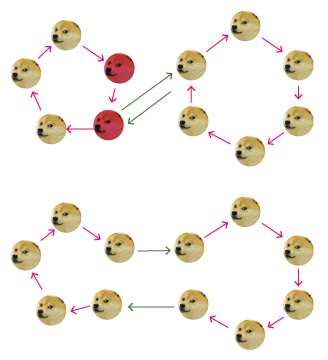# How Many Good Permutations?

Probability Level 5A permutation $(a_1, a_2, \cdots , a_{2014})$ of $(1, 2, \cdots , 2014)$ is called good if for all positive integers $k \leq 2014,$ $2 (a_1 + a_2 + \cdots + a_k)$ is a multiple of $k.$ Find the last three digits of the number of good permutations of $(1, 2, \cdots , 2014).$

Details and assumptions

• The general condition is $k \mid \displaystyle 2 \displaystyle \sum_{i=1}^{k} a_i$ for all positive integers $k \leq 2014.$

• This problem is not original.

×